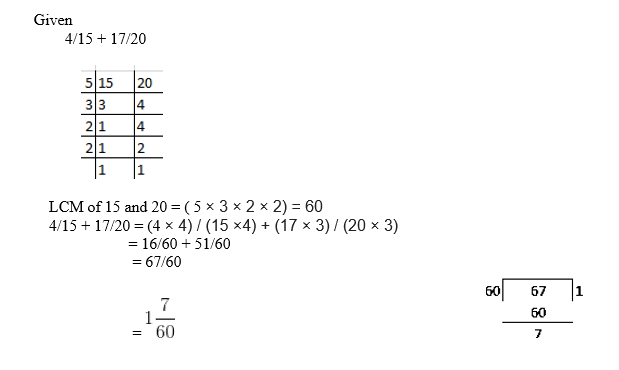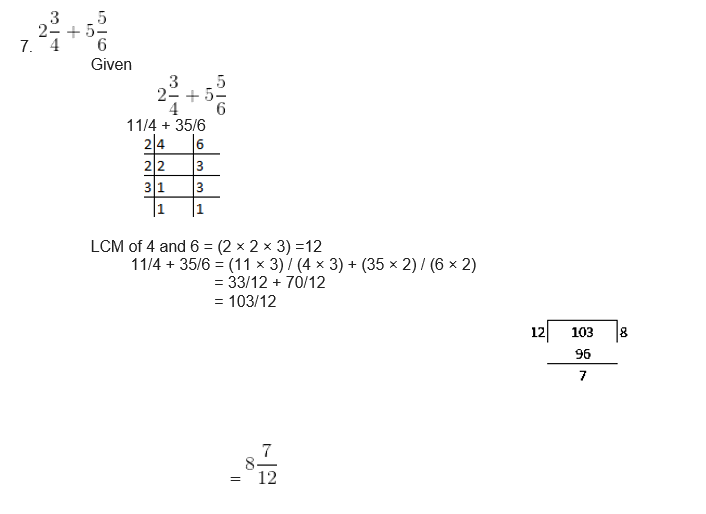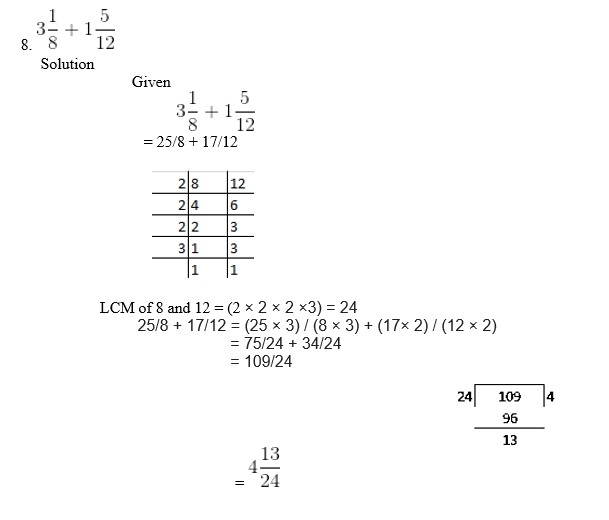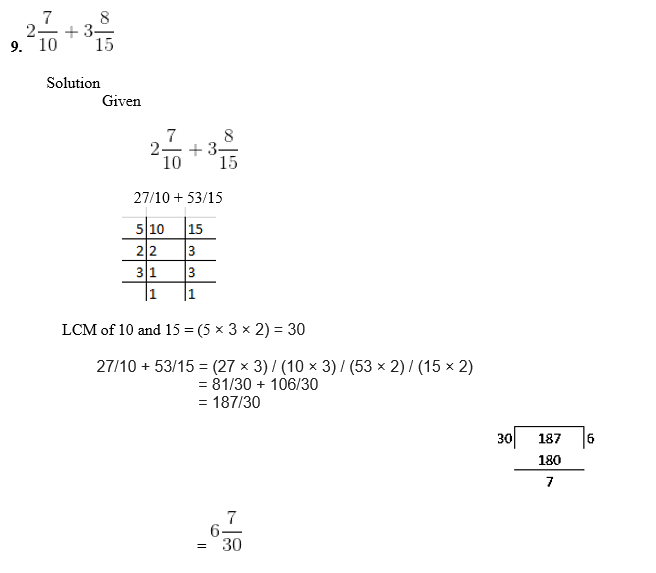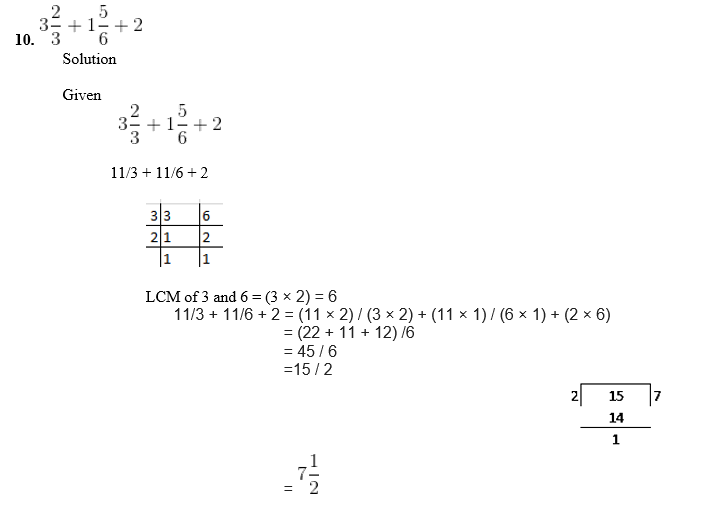# RS Aggarwal Solutions for Class 6 Chapter 5 Fractions Exercise 5E

RS Aggarwal Solutions for Class 6 Chapter 5 Fractions Exercise 5E are available here in PDF for students to boost their exam preparation. This exercise deals with the concepts like Addition of Like and Unlike Fractions along with solved examples. These solutions are a perfect reference guide for the students who face difficulty in solving problems and clear their doubts. BYJU’S experts prepare solutions of RS Aggarwal textbook to help students ace the exam. The students can download PDF from their respective links.

## Download PDF of RS Aggarwal Solutions for Class 6 Chapter 5 Fractions Exercise 5E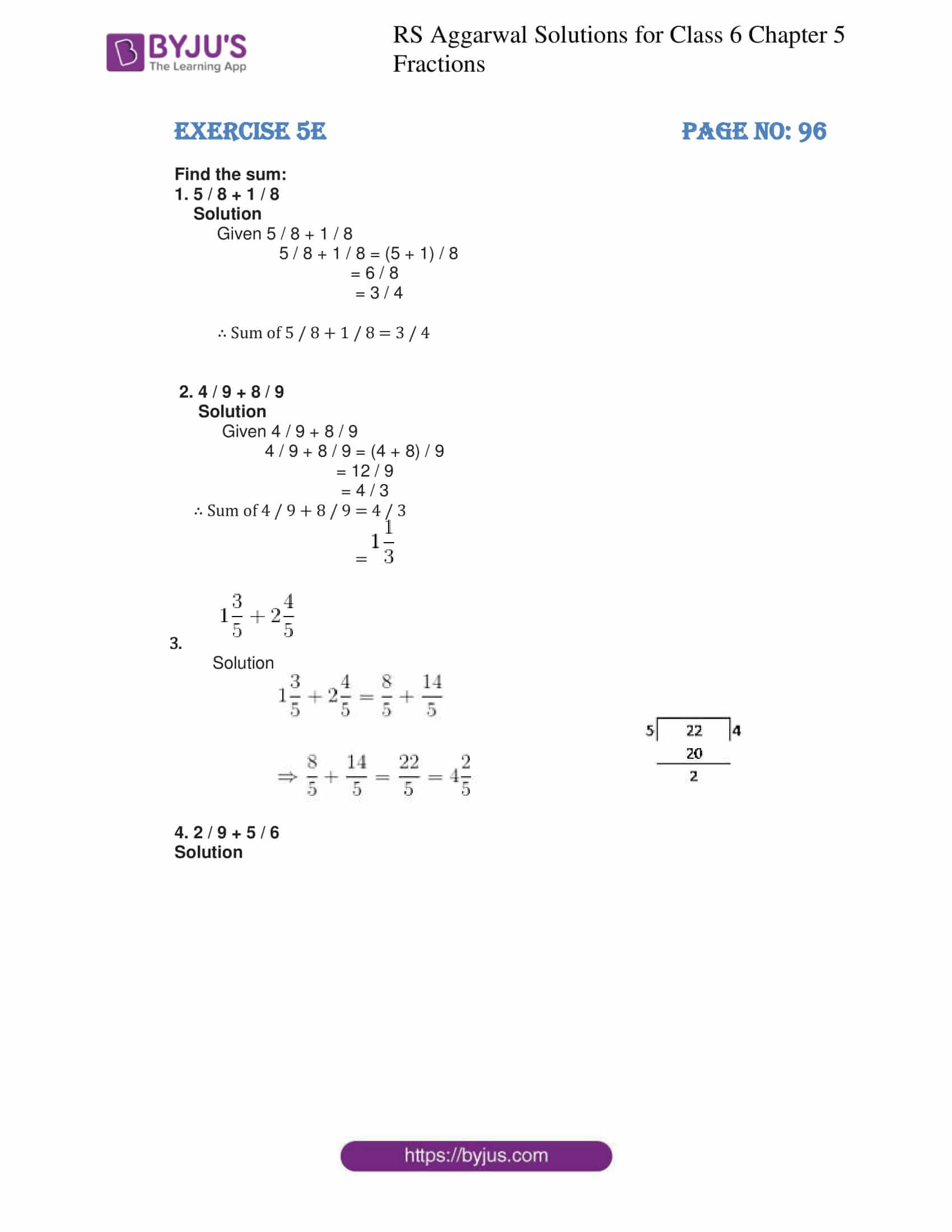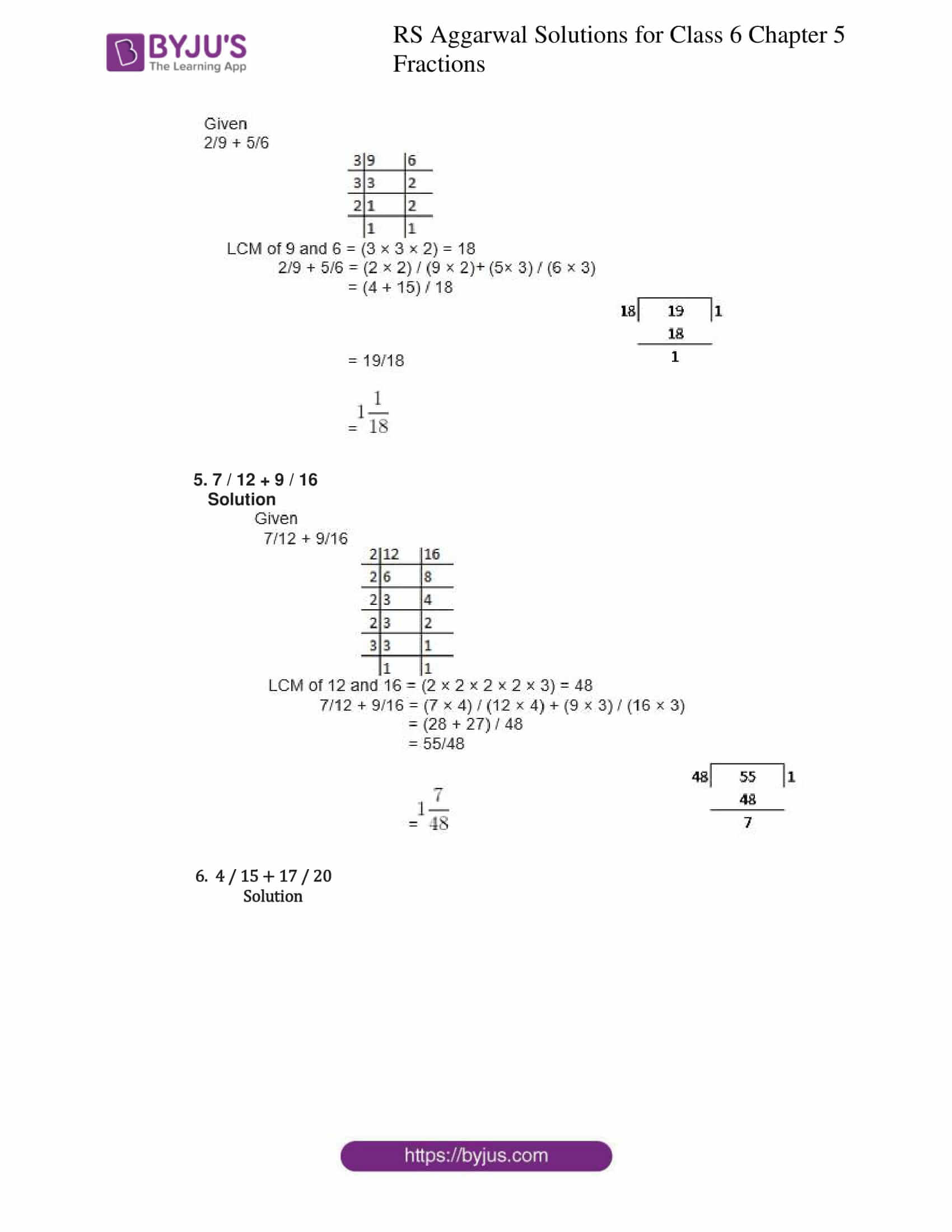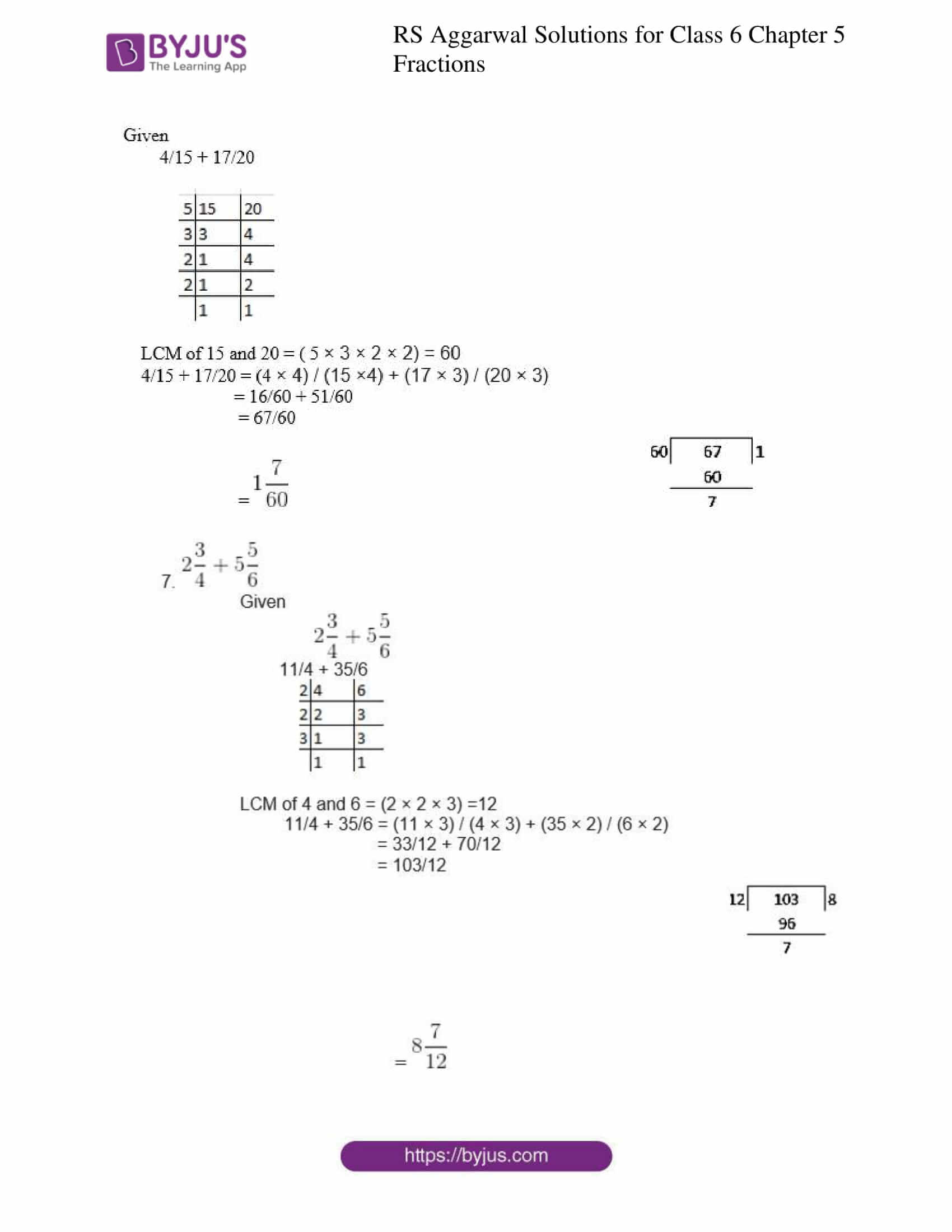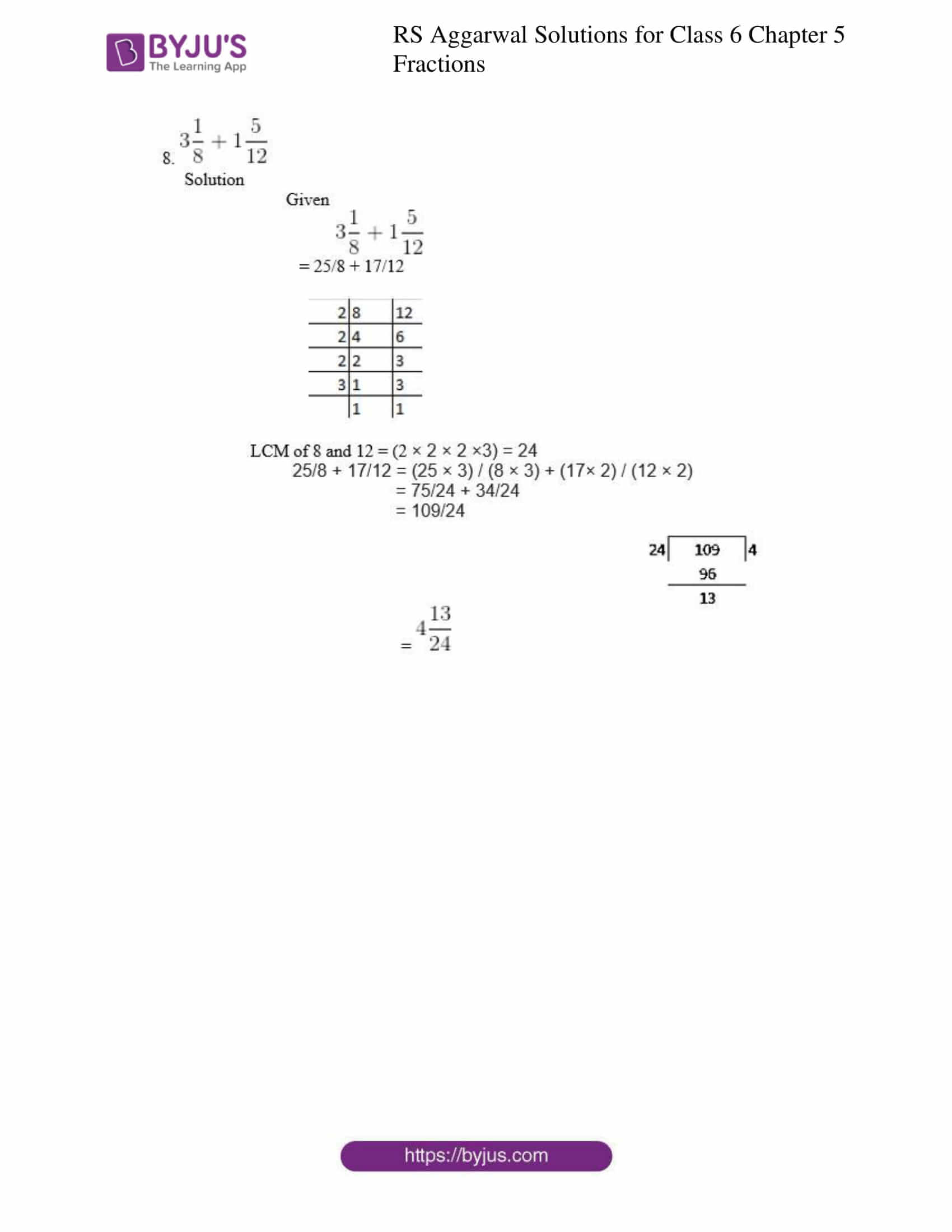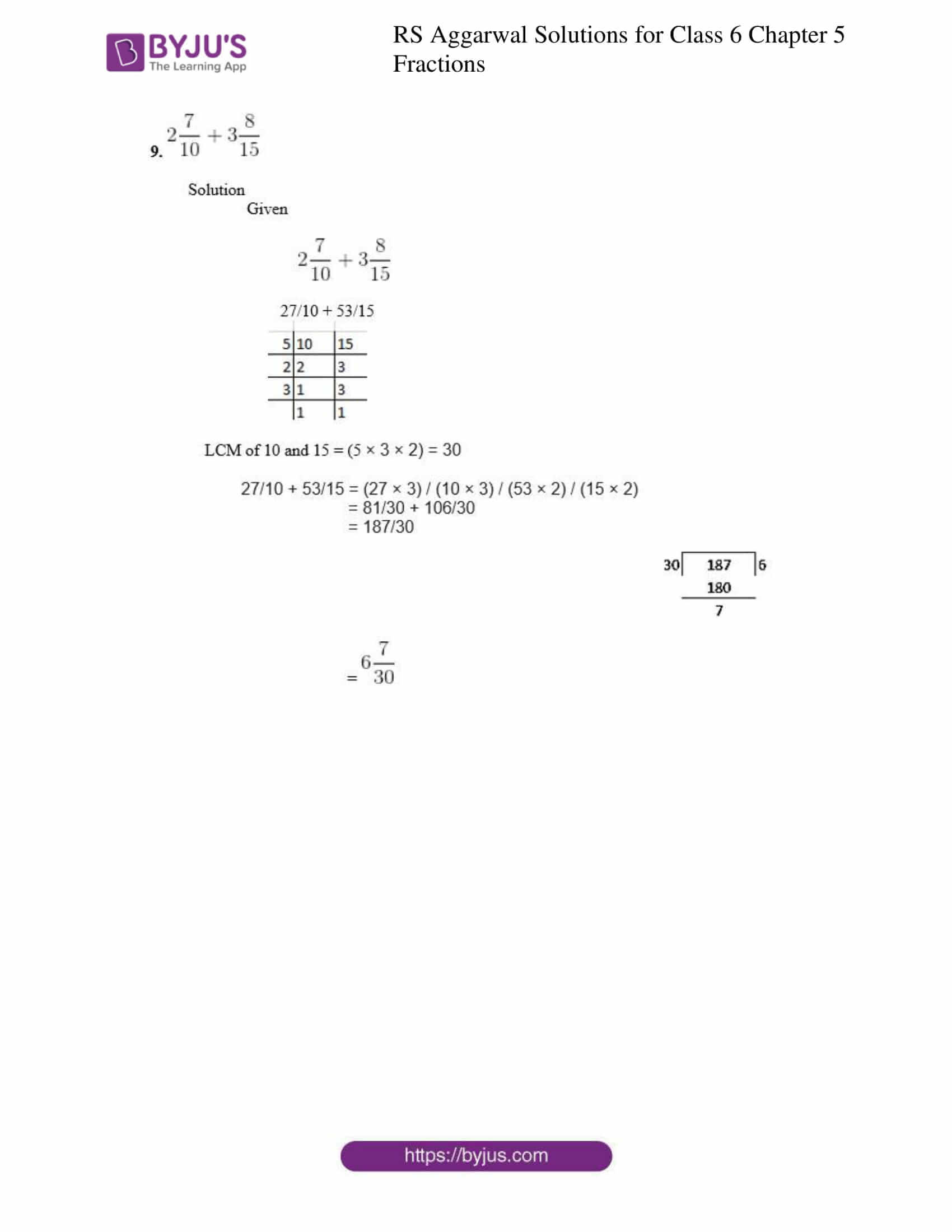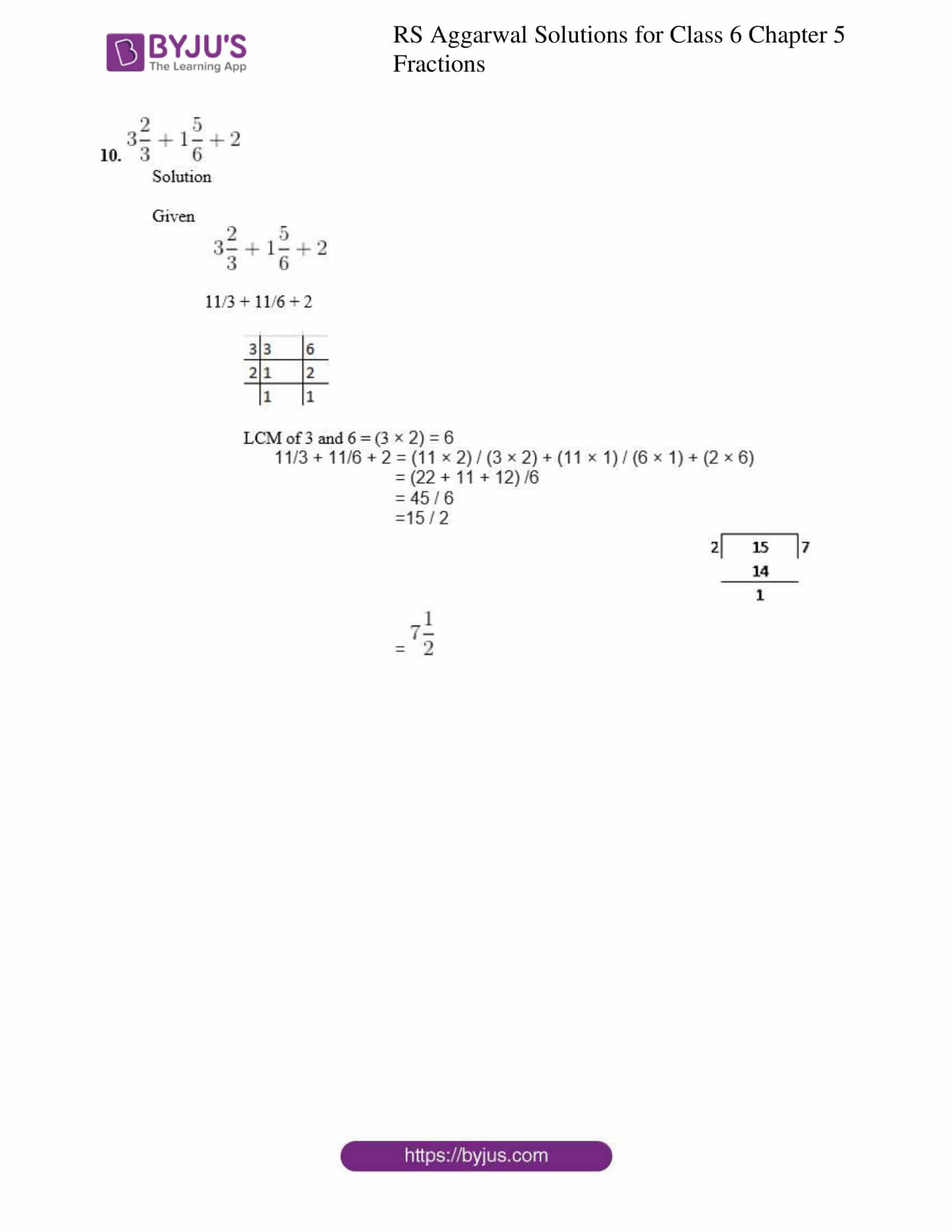### Access answers to Maths RS Aggarwal Solutions for Class 6 Chapter 5 Fractions Exercise 5E

Find the sum:

1. 5 / 8 + 1 / 8

Solution

Given 5 / 8 + 1 / 8

5 / 8 + 1 / 8 = (5 + 1) / 8

= 6 / 8

= 3 / 4

∴ Sum of 5 / 8 + 1 / 8 = 3 / 4

2. 4 / 9 + 8 / 9

Solution

Given 4 / 9 + 8 / 9

4 / 9 + 8 / 9 = (4 + 8) / 9

= 12 / 9

= 4 / 3

∴ Sum of 4 / 9 + 8 / 9 = 4 / 3

=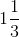3.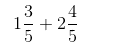Solution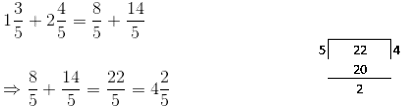4. 2 / 9 + 5 / 6

Solution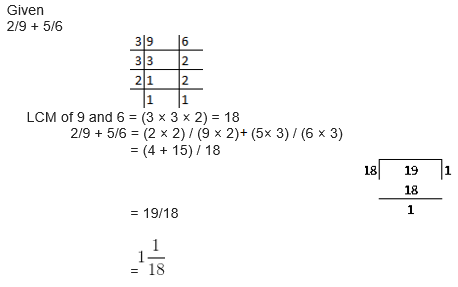5. 7 / 12 + 9 / 16

Solution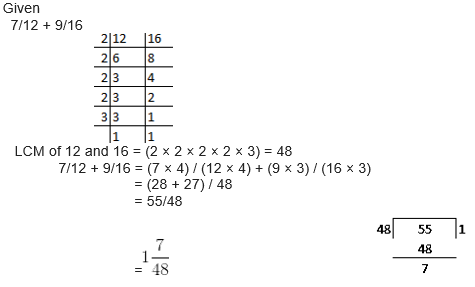6. 4 / 15 + 17 / 20

Solution